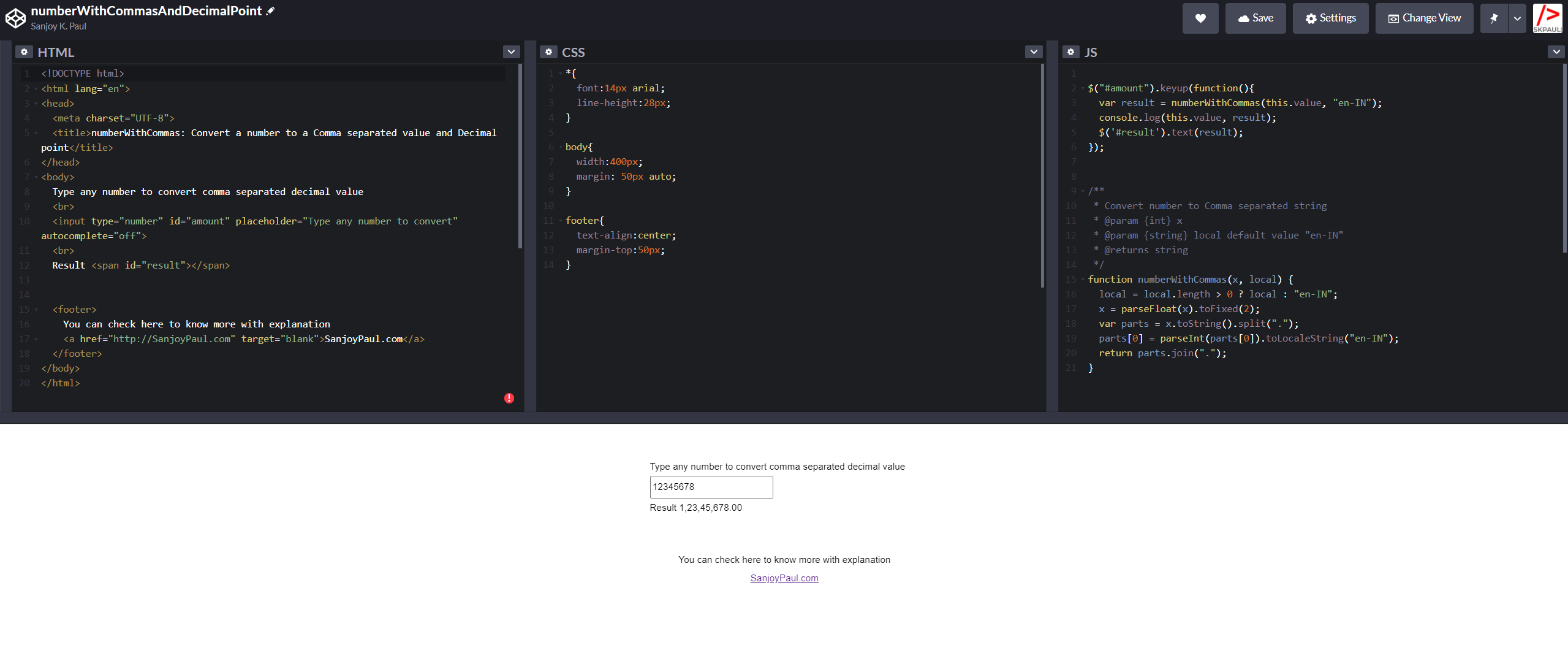# Convert a number to a Comma separated value or string with Decimal point in JavaScript``````/**
* Convert number to Comma separated decimal value
* @param {int} x
* @param {string} local default value "en-IN"
* @returns string
*/
function numberWithCommasAndDecimalPoint(x, local) {
local = local.length > 0 ? local : "en-IN";
x = parseFloat(x).toFixed(2);
var parts = x.toString().split(".");
parts = parseInt(parts).toLocaleString("en-IN");
return parts.join(".");
}``````

Let’s take a look line by line at what the above code does

local = local.length > 0 ? local : “en-IN”;

Checking the local parameter is empty or not. If it is empty, then set value “en-IN” otherwise set the provided local value.

x = parseFloat(x).toFixed(2);

Converting the provided number into float with parseFloat() function

to add decimal point to 2 digits by toFixed(2).

If you want to show 3 digits after decimal point you can pass 3 as argument like below

x.toFixed(3)

input: 1234.445500 output: 1234.445

var parts = x.toString().split(“.”);

toString() converts the number to string and split() function is splitting the value and make a array.

parts = parseInt(parts).toLocaleString(“en-IN”);

parseInt() converts the string to number. We are converting it to number again because we want to make a comma separated number by using JavaScript method or function toLocaleString() and passing “en-IN” because we want to present the number as Bangladeshi style (India and Bangladesh present the numbers same way). You can also any supported parameters from here.

return parts.join(“.”);

And at last joining the both parts (comma separated value and decimal value) through the join() function.

You can check an example here

This site uses Akismet to reduce spam. Learn how your comment data is processed.